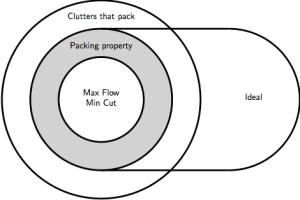# Clutters II

The last time I wrote on this subject, I made the case that clutters are of fairly natural interest to matroid theorists, since they are combinatorial objects with a duality involution, along with operations of deletion and contraction that are swapped by duality. They also arise fairly naturally in integer programming. In one formulation, integer programming is the problem of finding an integral vector $x$ that minimises the quantity $w^{T}x$ ($w$ is a fixed vector of weights) subject to the constraints $x\geq \mathbf{0}$ and $Mx\geq b$, where $M$ is a matrix of constraints. (Every entry of $\mathbf{0}$ is zero, and all comparisons of vectors are done entrywise – $x\geq \mathbf{0}$ means that every entry of $x$ is non-negative.)

Many optimisation problems in combinatorics can be expressed as integer programs. In particular, several problems are equivalent to integer programs where $b$ is equal to the vector of all ones, and $M$ contains only zeroes and ones. It’s an easy exercise to show that finding a minimum-size vertex cover in a graph is one of these problems. So is finding a minimum-size $s$-$t$ cut in a network.

In these cases, we may as well assume that the support of no row in $M$ properly contains the support of another row: if $r$ and $r’$ are rows of $M$, and $r$ is equal to $1$ in every position that $r’$ is equal to $1$, then we might as well remove the row $r$. This does not change the solution space of the program at all. In other words, we may as well assume that the rows of $M$ are the characteristic vectors of the edges in a clutter.

So clutters arise from combinatorial optimisation problems, via integer programming, and this leads to the following Venn diagram.My aim today is modest: to define these classes of clutters. The illustration, along with essentially all the content found here, is in Combinatorial Optimisation: Packing and Covering, by Gérards Cornuéjols.

First of all, let $H=(S,\mathcal{A})$ be a clutter, so that $S$ is a finite set, and the members of $\mathcal{A}$ are subsets of $S$, none of which is properly contained in another. Let $M(H)$ be the incidence matrix of $H$. That is, the columns of $M(H)$ are labelled by the elements of $S$, and the rows are labelled by members of $\mathcal{A}$. An entry of $M(H)$ is one if and only if the corresponding element of $S$ is contained in the corresponding member of $\mathcal{A}$, otherwise it is zero.

Now assume that $w$ and $x$ are $|S|\times 1$ vectors with rows labelled by elements of $S$ (in the same order as the columns of $M(H)$), and the entries of $w$ non-negative. The vector $w$ can be seen as assigning a weight to each element of $S$. We will consider the following linear program.

(1) Minimise $w^{T}x$ subject to the constraints $x\geq \mathbf{0}$ and $M(H)x\geq \mathbf{1}$.

(Here $\mathbf{1}$ is the vector of all ones.)

If $x$ is an integer solution to this problem, then there is no harm in assuming that the entries of $x$ are all $0$ or $1$. (If any entry is greater than $1$, then replace it with $1$. Then $M(H)x\geq \mathbf{1}$ will still hold, and $w^{T}x$ will not have increased.) So an integer solution can be seen as a characteristic vector of a subset of $S$. This subset must intersect every member of $\mathcal{A}$ (by virtue of the constraint $M(H)x\geq \mathbf{1}$). Thus we are looking for a minimum-weight hitting set, and (1) can be thought of as the relaxation of the hitting set problem that allows non-integral solutions.

Next we consider the dual problem. Here $y$ is a $|\mathcal{A}|\times 1$ vector with entries labelled by the members of $\mathcal{A}$.

(2) Maximise $y^{T}\mathbf{1}$ subject to the constraints $y\geq \mathbf{0}$ and $y^{T}M(H)\leq w$.

In this problem we are thinking of $w$ as a capacity on each element of $S$. The vector $y$ assigns a non-negative flow value to each edge in $\mathcal{A}$, and the constraint $y^{T}M(H)\leq w$ says that the sum of flows over the edges that contain an element $s\in S$ cannot exceed the capacity that $w$ assigns to $s$. A solution to (2) maximises the sum of the flow values.

Now we can define the four classes in the Venn diagram.

$H$ has the Max Flow Min Cut property if, for every non-negative integer vector $w$, the programs (1) and (2) both have optimal solutions, $x$ and $y$, that are integral.

$H$ has the packing property if, for every vector $w$ whose entries are $0$, $1$, or $+\infty$, the programs (1) and (2) both have optimal solutions that are integral. In program (1), a weight of $+\infty$ means that we try to never include that element of $S$ in our hitting set. A weight of $0$ means that we can include that element for free.

$H$ packs if programs (1) and (2) have optimal solutions that are integral vectors when $w$ is set to be $\mathbf{1}$.

$H$ is ideal if, for any choice of vector $w$ with non-negative entries, not necessarily integral, program (1) has an optimal solution that is an integral vector.

Next time I will say more about the relations between these properties. For now, I will just note that, rather unfortunately, there really are clutters that pack, but which do not have the packing property. On the other hand, the shaded area of the Venn diagram is believed to be empty. So it is conjectured that a clutter has the Max Flow Min Cut property if and only if it has the packing property. Cornuéjols offers a bounty of \$5000 for a resolution of this conjecture (or one of 17 others) before 2020.

This site uses Akismet to reduce spam. Learn how your comment data is processed.